Successfully reported this slideshow.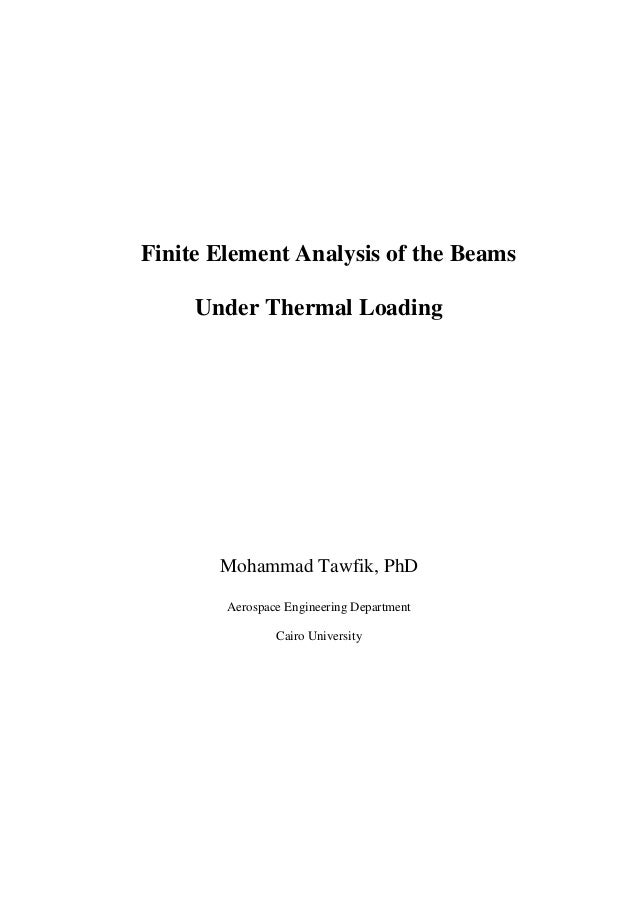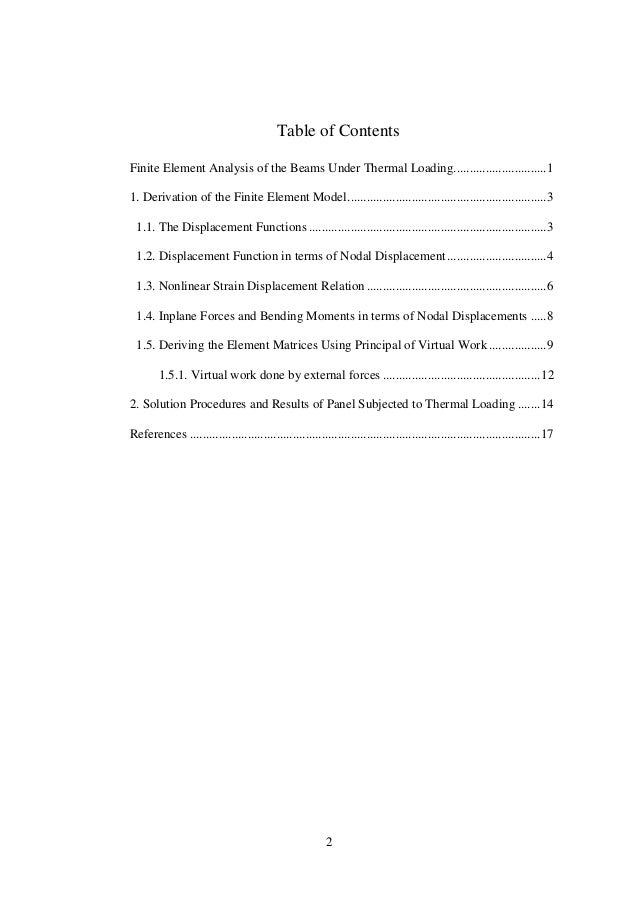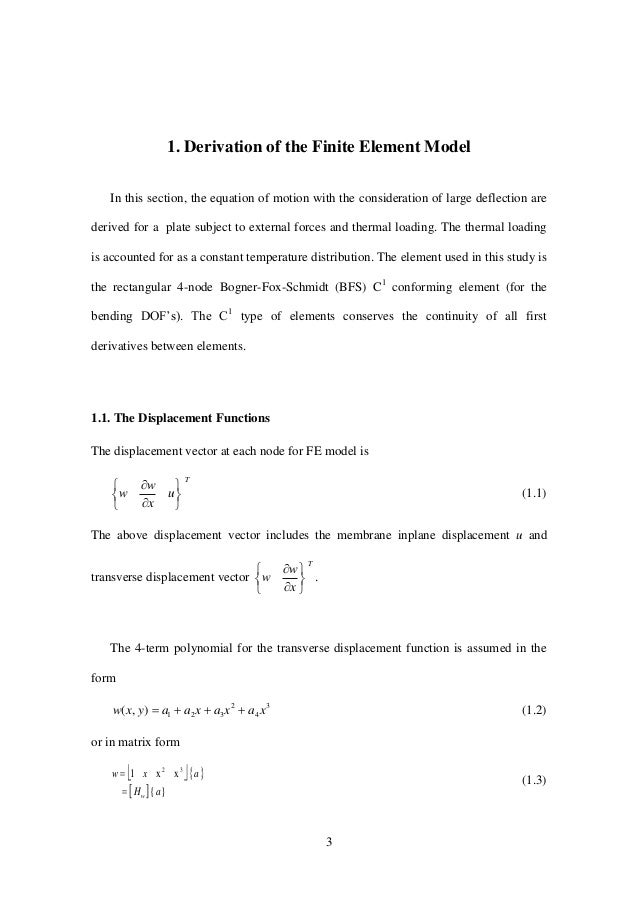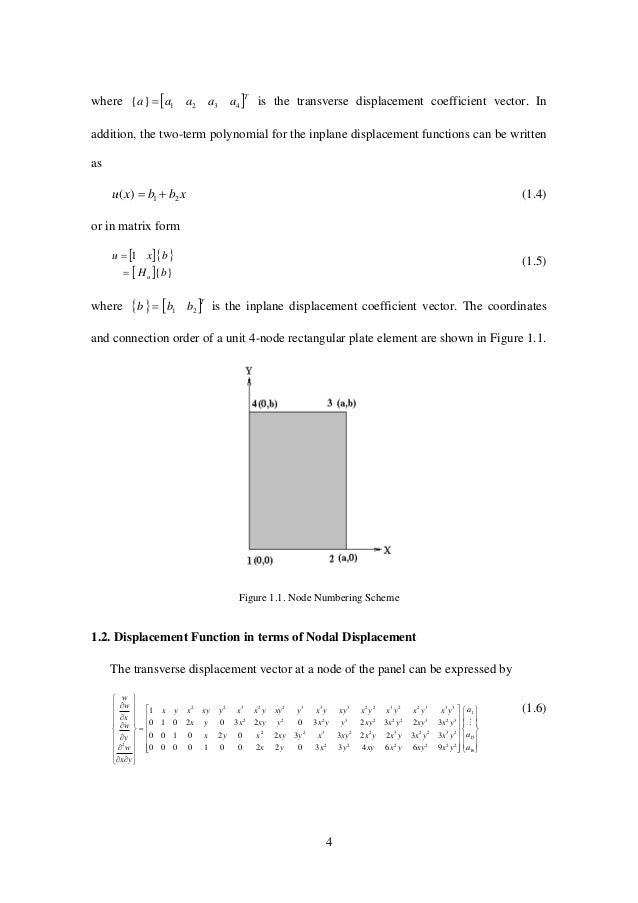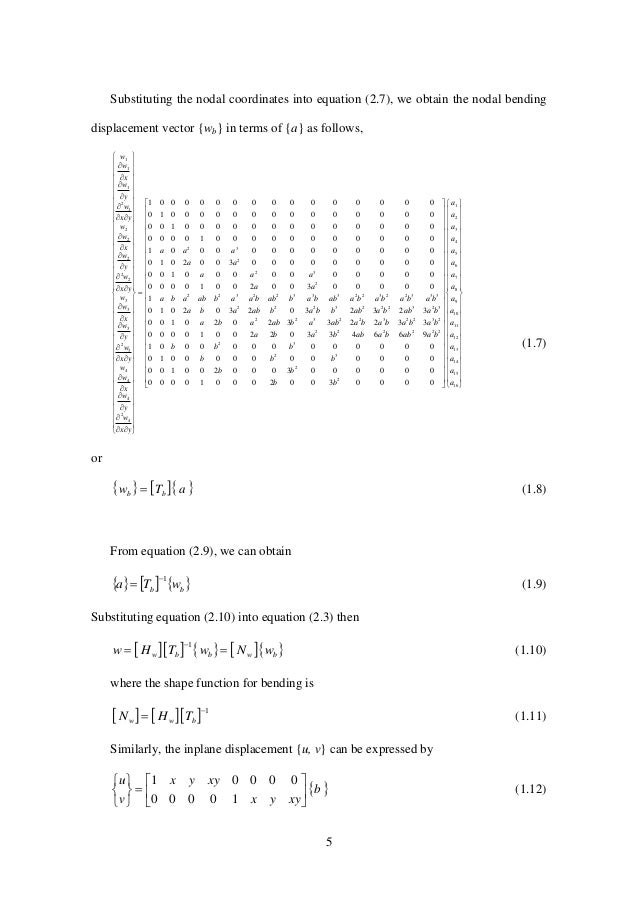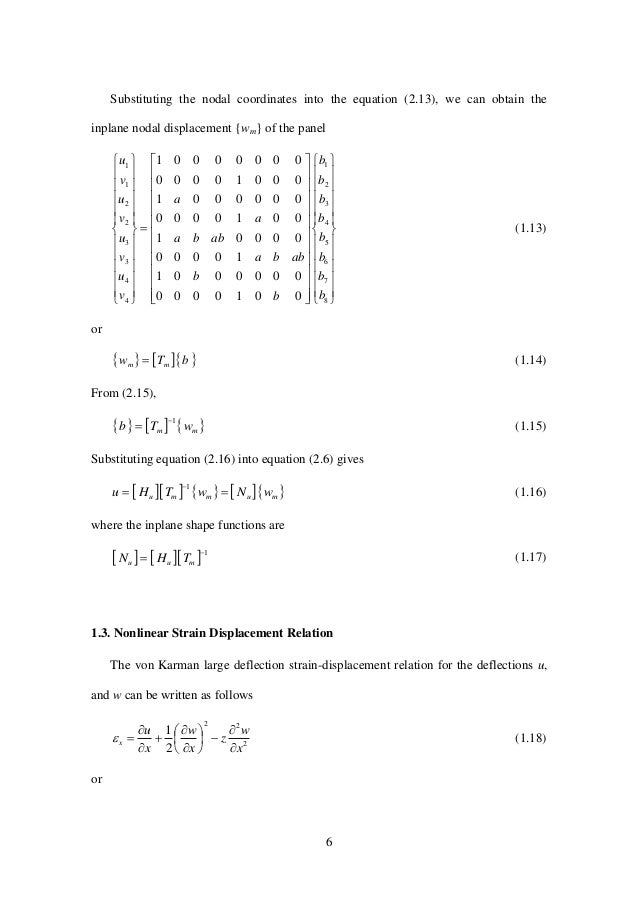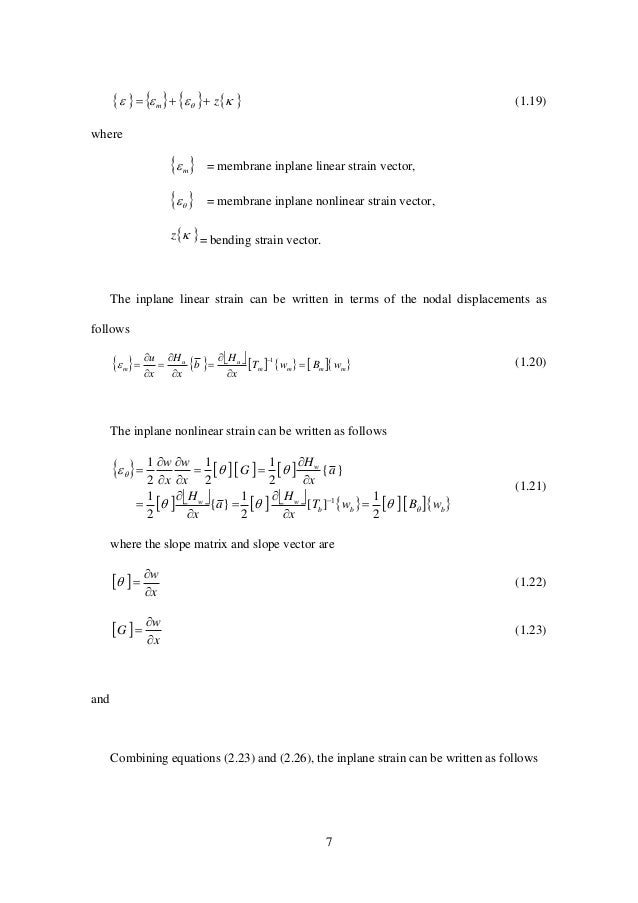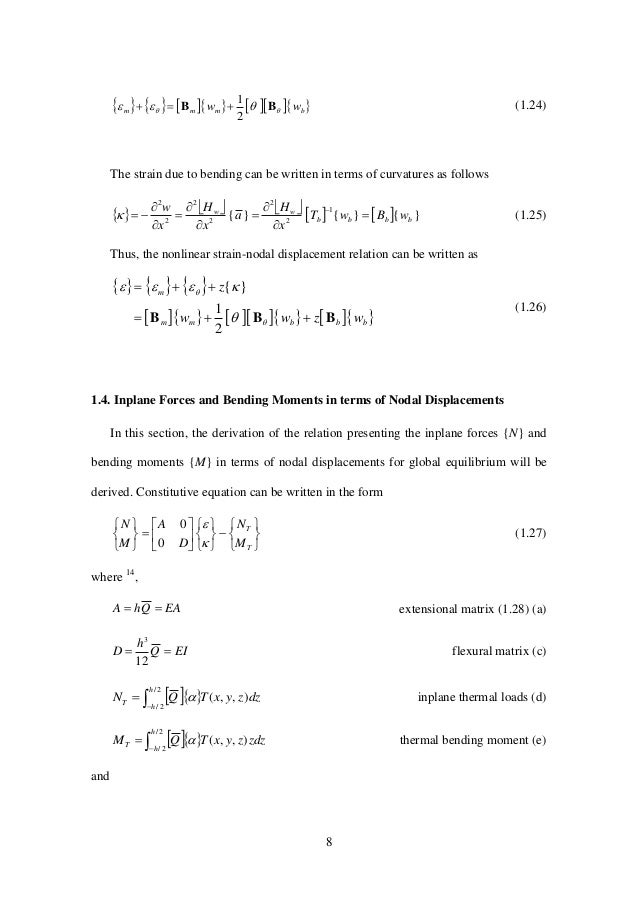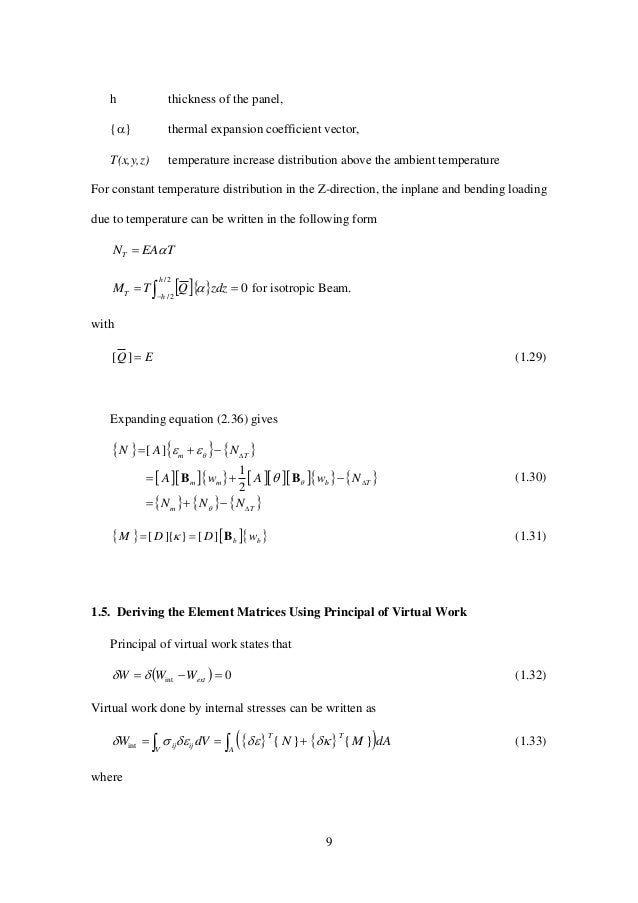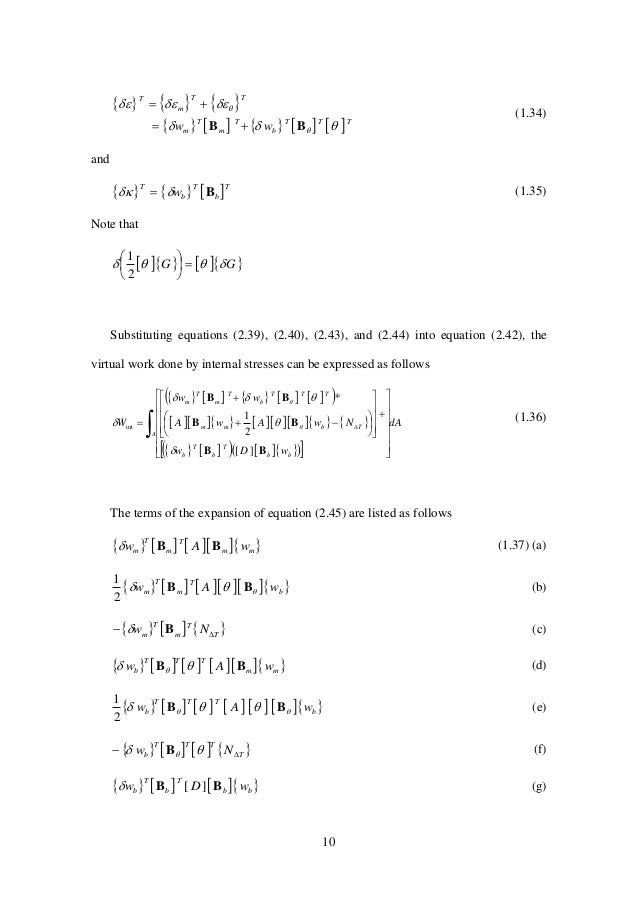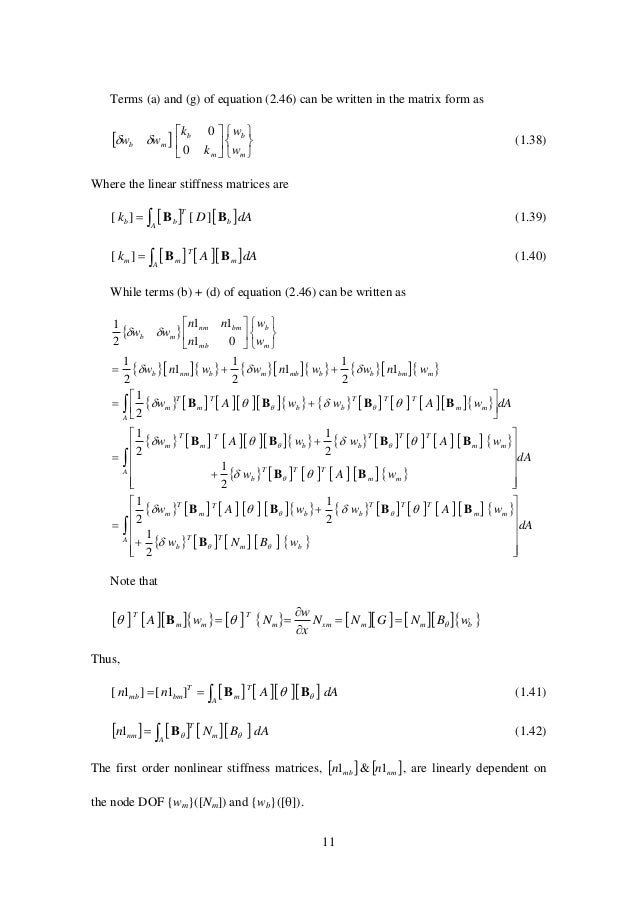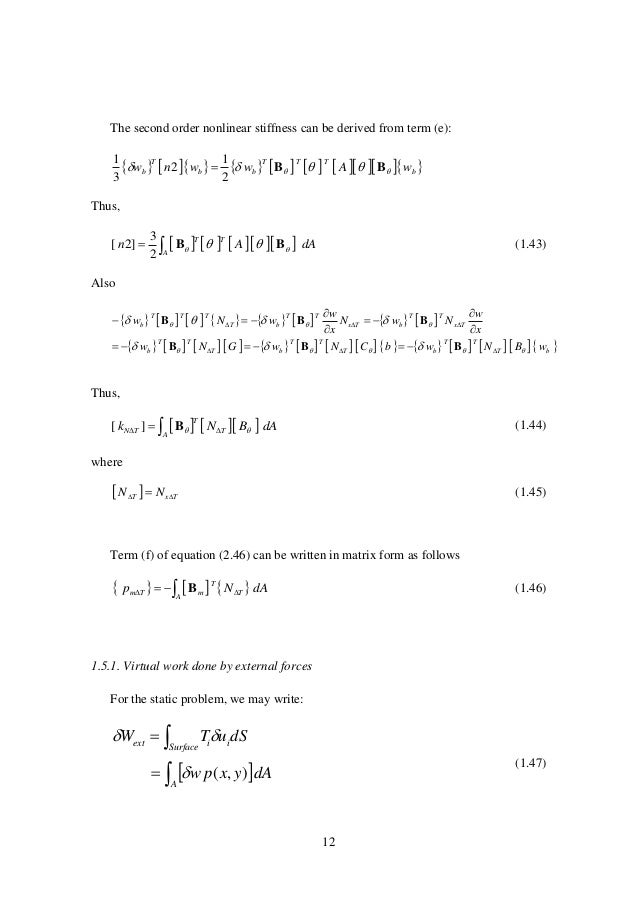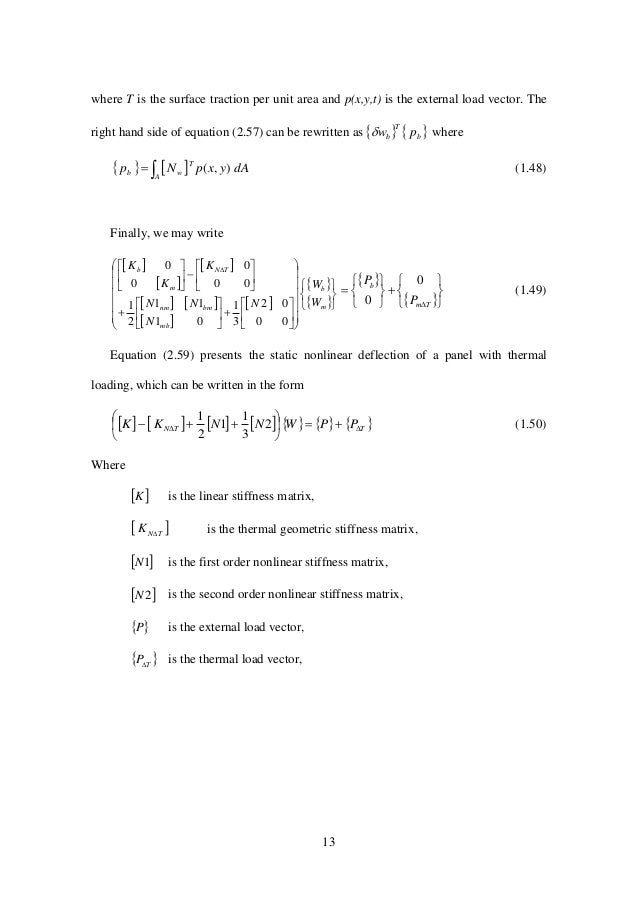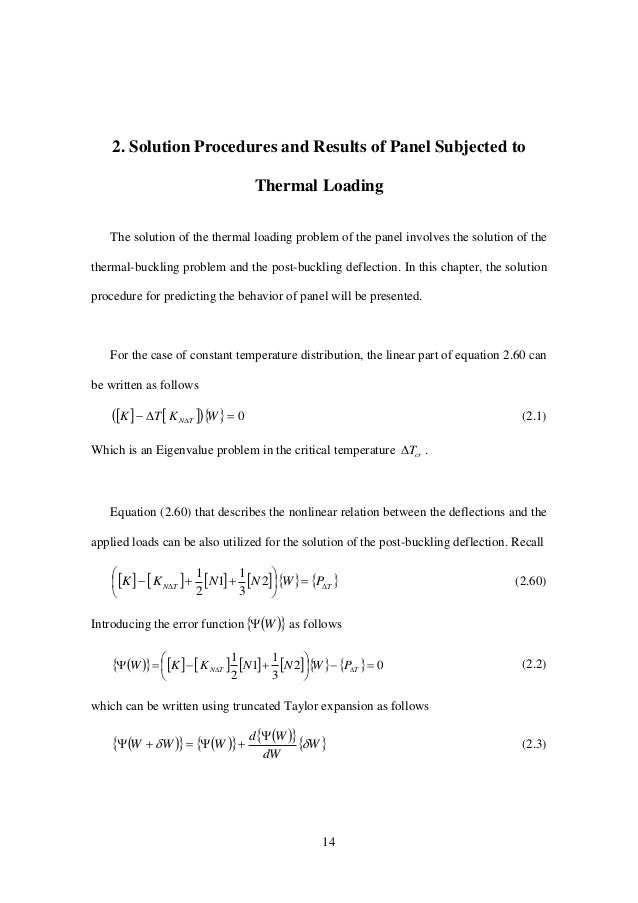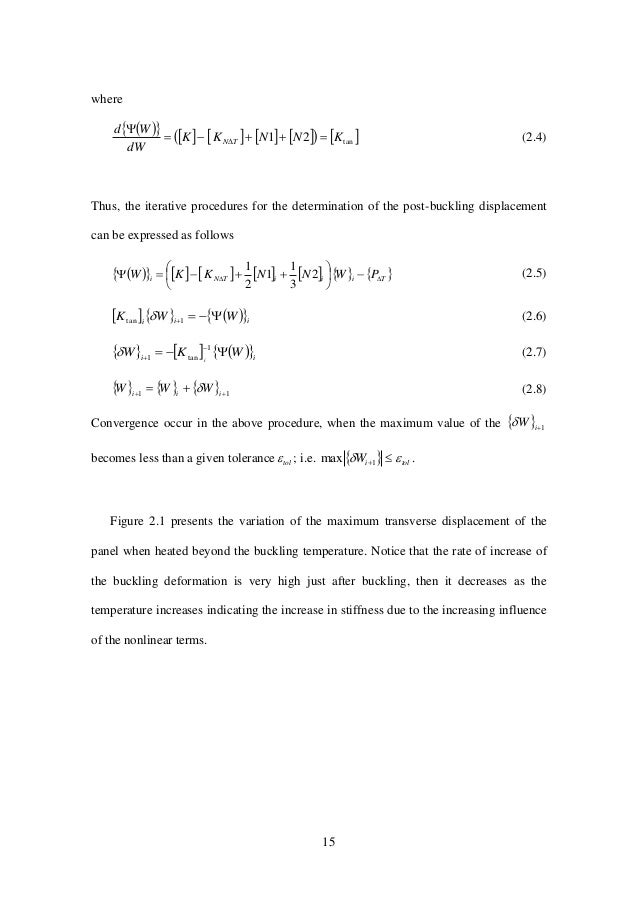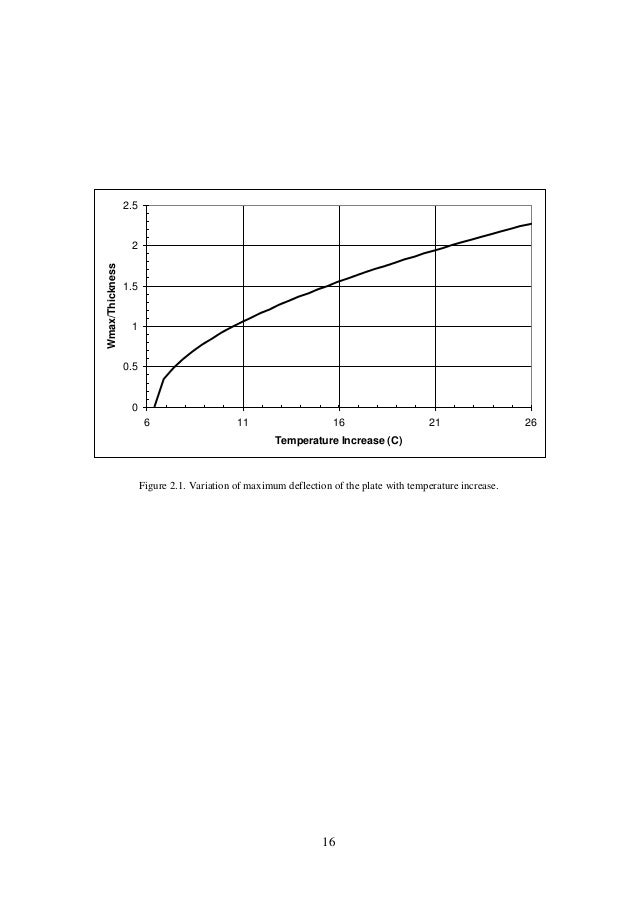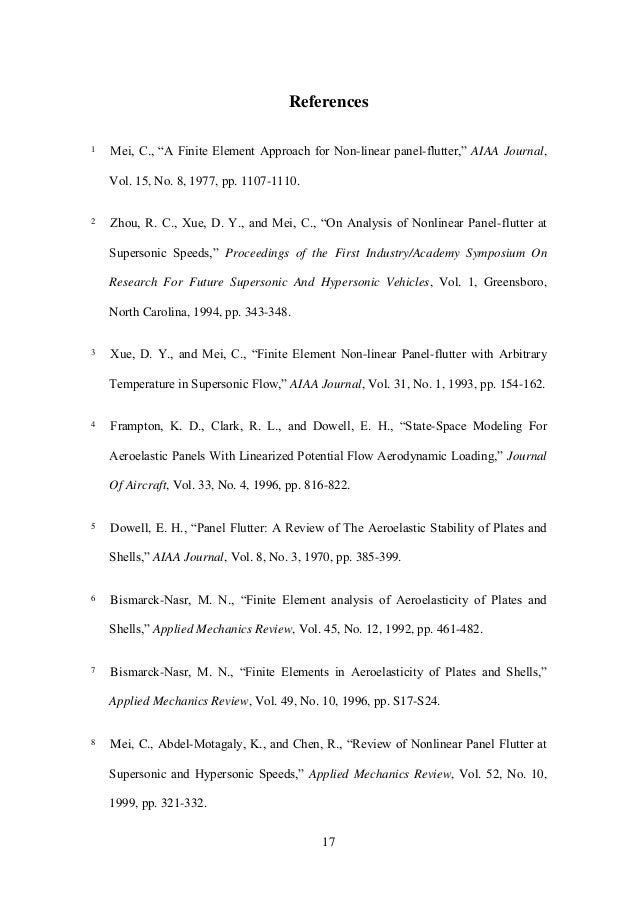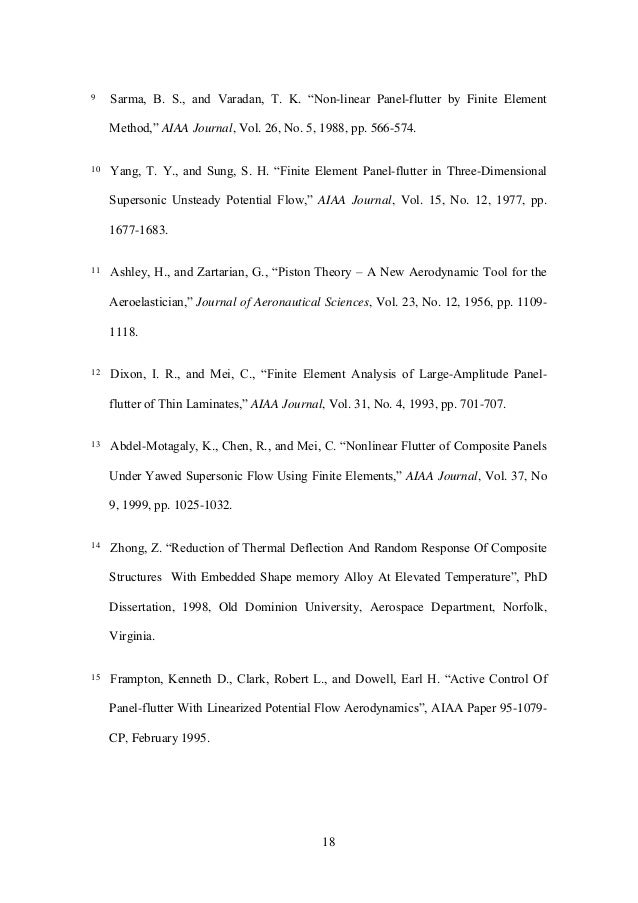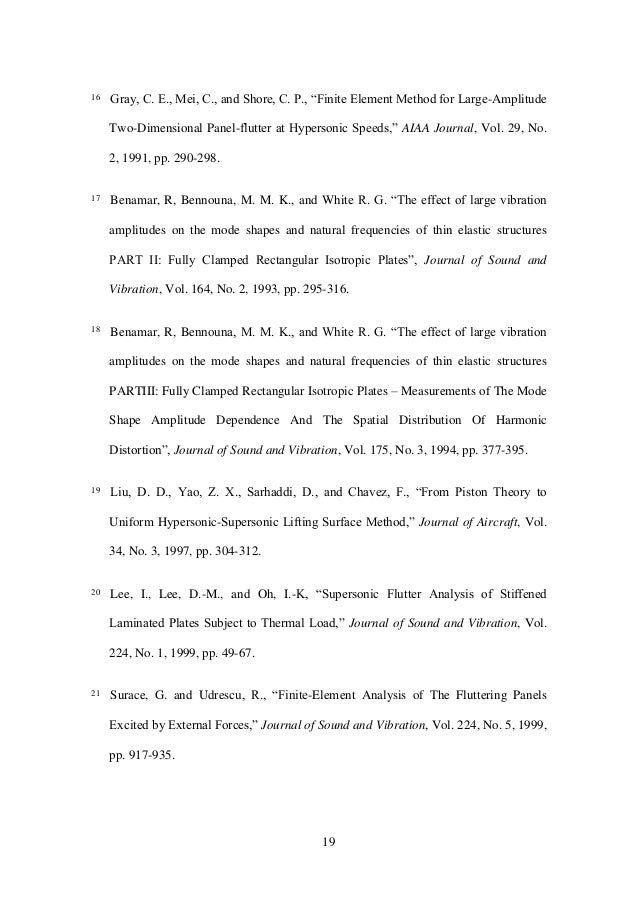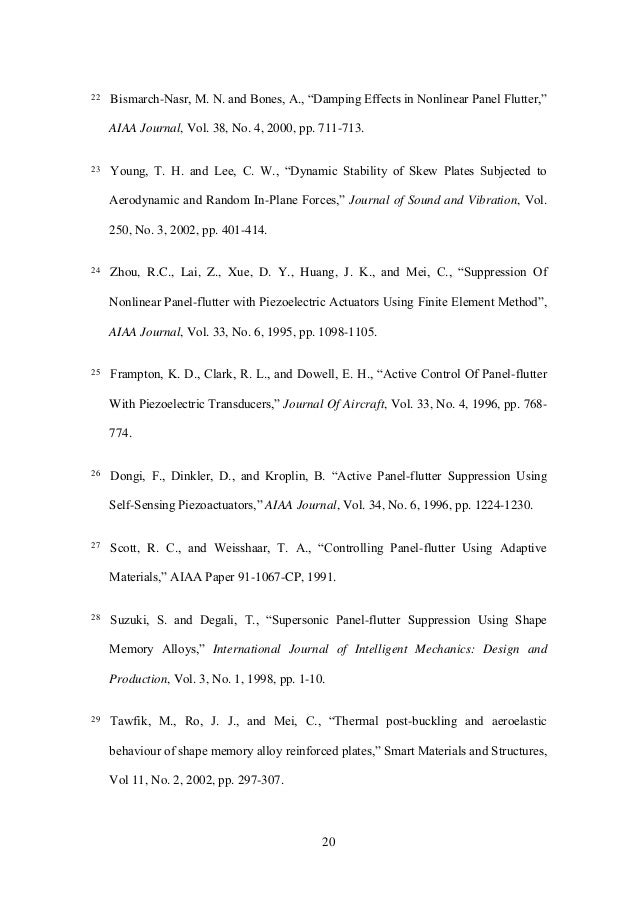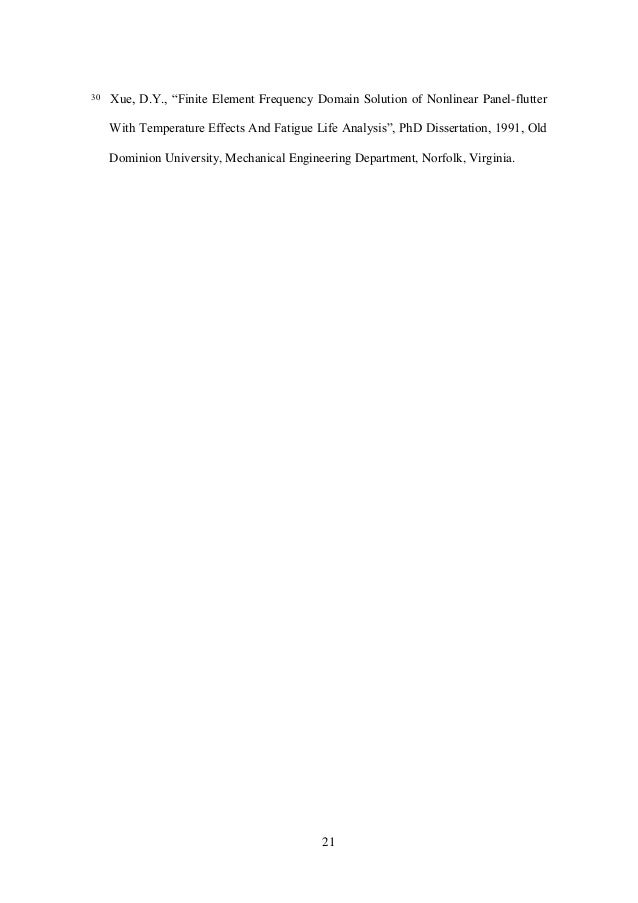Upcoming SlideShare
×

A report on the finite element analysis of a beam under thermal loading. Nonlinear deflections and solution procedures covered.

#WikiCourses
https://wikicourses.wikispaces.com/TopicX+Nonlinear+Solid+Mechanics
https://eau-esa.wikispaces.com/Topic+Nonlinear+Solid+Mechanics

• Full Name
Comment goes here.

Are you sure you want to Yes No• Be the first to comment

1. 1. Finite Element Analysis of the Beams Under Thermal Loading Mohammad Tawfik, PhD Aerospace Engineering Department Cairo University
2. 2. 2 Table of Contents Finite Element Analysis of the Beams Under Thermal Loading.............................1 1. Derivation of the Finite Element Model..............................................................3 1.1. The Displacement Functions ..........................................................................3 1.2. Displacement Function in terms of Nodal Displacement...............................4 1.3. Nonlinear Strain Displacement Relation........................................................6 1.4. Inplane Forces and Bending Moments in terms of Nodal Displacements .....8 1.5. Deriving the Element Matrices Using Principal of Virtual Work..................9 1.5.1. Virtual work done by external forces .................................................12 2. Solution Procedures and Results of Panel Subjected to Thermal Loading .......14 References .............................................................................................................17
3. 3. 3 1. Derivation of the Finite Element Model In this section, the equation of motion with the consideration of large deflection are derived for a plate subject to external forces and thermal loading. The thermal loading is accounted for as a constant temperature distribution. The element used in this study is the rectangular 4-node Bogner-Fox-Schmidt (BFS) C1 conforming element (for the bending DOF’s). The C1 type of elements conserves the continuity of all first derivatives between elements. 1.1. The Displacement Functions The displacement vector at each node for FE model is T u x w w         (1.1) The above displacement vector includes the membrane inplane displacement u and transverse displacement vector T x w w         . The 4-term polynomial for the transverse displacement function is assumed in the form 3 4 2 321),( xaxaxaayxw  (1.2) or in matrix form      }{ xx1 32 aH axw w  (1.3)
4. 4. 4 where  T aaaaa 4321}{  is the transverse displacement coefficient vector. In addition, the two-term polynomial for the inplane displacement functions can be written as xbbxu 21)(  (1.4) or in matrix form      }{ 1 bH bxu u  (1.5) where    T bbb 21 is the inplane displacement coefficient vector. The coordinates and connection order of a unit 4-node rectangular plate element are shown in Figure 1.1. Figure 1.1. Node Numbering Scheme 1.2. Displacement Function in terms of Nodal Displacement The transverse displacement vector at a node of the panel can be expressed by                                                      16 15 1 222222 2322322322 3232223222 3332232233322322 2 9664330220010000 3322332020100 3232302302010 1 a a a yxxyyxxyyxyx yxyxyxyxxyxyxyxyx yxxyyxxyyyxyxyxyx yxyxyxyxxyyxyxyyxxyxyxyx yx w y w x w w  (1.6)
5. 5. 5 Substituting the nodal coordinates into equation (2.7), we obtain the nodal bending displacement vector {wb} in terms of {a} as follows,                                                                                                                                                                                                        16 15 14 13 12 11 10 9 8 7 6 5 4 3 2 1 2 2 32 32 222222 2322322322 3232223222 3332232233322322 2 32 2 32 4 2 4 4 4 3 2 3 3 3 2 2 2 2 2 1 2 1 1 1 0000300200010000 0000003000200100 0000000000010 0000000000001 9664330220010000 3322332020100 3232302302010 1 0000030020010000 0000000000100 0000000003002010 0000000000001 0000000000010000 0000000000000100 0000000000000010 0000000000000001 a a a a a a a a a a a a a a a a bb bb bbb bbb baabbaabbaba babababaababababa baabbaabbbabababa babababaabbababbaabababa aa aaa aa aaa yx w y w x w w yx w y w x w w yx w y w x w w yx w y w x w w (1.7) or     aTw bb  (1.8) From equation (2.9), we can obtain      bb wTa 1  (1.9) Substituting equation (2.10) into equation (2.3) then        bwbbw wNwTHw  1 (1.10) where the shape function for bending is      1  bww THN (1.11) Similarly, the inplane displacement {u, v} can be expressed by  b xyyx xyyx v u              10000 00001 (1.12)
6. 6. 6 Substituting the nodal coordinates into the equation (2.13), we can obtain the inplane nodal displacement {wm} of the panel                                                                                8 7 6 5 4 3 2 1 4 4 3 3 2 2 1 1 0010000 0000001 10000 00001 0010000 0000001 00010000 00000001 b b b b b b b b b b abba abba a a v u v u v u v u (1.13) or     bTw mm  (1.14) From (2.15),      mm wTb 1  (1.15) Substituting equation (2.16) into equation (2.6) gives        mummu wNwTHu  1 (1.16) where the inplane shape functions are      1  muu THN (1.17) 1.3. Nonlinear Strain Displacement Relation The von Karman large deflection strain-displacement relation for the deflections u, and w can be written as follows 2 22 2 1 x w z x w x u x               (1.18) or
7. 7. 7          zm  (1.19) where  m = membrane inplane linear strain vector,   = membrane inplane nonlinear strain vector,  z = bending strain vector. The inplane linear strain can be written in terms of the nodal displacements as follows            mmmm uu m wBwT x H b x H x u           1  (1.20) The inplane nonlinear strain can be written as follows                     bbb ww w wBwT x H a x H a x H G x w x w     2 1 ][ 2 1 }{ 2 1 }{ 2 1 2 1 2 1 1                 (1.21) where the slope matrix and slope vector are   x w    (1.22)   x w G    (1.23) and Combining equations (2.23) and (2.26), the inplane strain can be written as follows
8. 8. 8           bmmm ww   BB 2 1  (1.24) The strain due to bending can be written in terms of curvatures as follows           }{}{}{ 1 2 2 2 2 2 2 bbbb ww wBwT x H a x H x w             (1.25) Thus, the nonlinear strain-nodal displacement relation can be written as                bbbmm m wzww z BBB       2 1 }{ (1.26) 1.4. Inplane Forces and Bending Moments in terms of Nodal Displacements In this section, the derivation of the relation presenting the inplane forces {N} and bending moments {M} in terms of nodal displacements for global equilibrium will be derived. Constitutive equation can be written in the form                           T T M N D A M N   0 0 (1.27) where 14 , EAQhA  extensional matrix (1.28) (a) EIQ h D  12 3 flexural matrix (c)     2/ 2/ ),,( h h T dzzyxTQN  inplane thermal loads (d)     2/ 2/ ),,( h h T zdzzyxTQM  thermal bending moment (e) and
9. 9. 9 h thickness of the panel, {} thermal expansion coefficient vector, T(x,y,z) temperature increase distribution above the ambient temperature For constant temperature distribution in the Z-direction, the inplane and bending loading due to temperature can be written in the following form TEANT     0 2/ 2/   h h T zdzQTM  for isotropic Beam. with EQ ][ (1.29) Expanding equation (2.36) gives                       Tm Tbmm Tm NNN NwAwA NAN            BB 2 1 ][ (1.30)     bb wDDM B][}]{[   (1.31) 1.5. Deriving the Element Matrices Using Principal of Virtual Work Principal of virtual work states that   0int  extWWW  (1.32) Virtual work done by internal stresses can be written as        V A TT ijij dAMNdVW }{}{int  (1.33) where
10. 10. 10                TTT b T m T m TT m T ww     BB   (1.34) and      T b T b T w B  (1.35) Note that      GG        2 1 Substituting equations (2.39), (2.40), (2.43), and (2.44) into equation (2.42), the virtual work done by internal stresses can be expressed as follows                                 dA wDw NwAwA ww W A bb T b T b Tbmm TTT b T m T m                                     BB BB BB ][ 2 1 * int       (1.36) The terms of the expansion of equation (2.45) are listed as follows        mm T m T m wAw BB (1.37) (a)         b T m T m wAw  BB 2 1 (b)      T T m T m Nw  B (c)          mm TTT b wAw BB   (d)             b TTT b wAw   BB 2 1 (e)        T TTT b Nw   B (f)       bb T b T b wDw BB ][ (g)
11. 11. 11 Terms (a) and (g) of equation (2.46) can be written in the matrix form as               m b m b mb w w k k ww 0 0  (1.38) Where the linear stiffness matrices are    dADk A b T bb  BB ][][ (1.39)     dAAk A m T mm  BB][ (1.40) While terms (b) + (d) of equation (2.46) can be written as                                                                                                                                                   A bm TT b mm TTT bb T m T m A mm TTT b mm TTT bb T m T m A mm TTT bb T m T m mbmbbmbmbnmb m b mb bmnm mb dA wBNw wAwwAw dA wAw wAwwAw dAwAwwAw wnwwnwwnw w w n nn ww             B BBBB BB BBBB BBBB 2 1 2 1 2 1 2 1 2 1 2 1 2 1 1 2 1 1 2 1 1 2 1 01 11 2 1 Note that                 bmmxmm T mm T wBNGNN x w NwA     B Thus,       dAAnn A T m T bmmb   BB]1[]1[ (1.41)        dABNn A m T nm  B1 (1.42) The first order nonlinear stiffness matrices,    nmmb nn 1&1 , are linearly dependent on the node DOF {wm}([Nm]) and {wb}([]).
12. 12. 12 The second order nonlinear stiffness can be derived from term (e):                b TTT bb T b wAwwnw   BB 2 1 2 3 1  Thus,         dAAn A TT    BB 2 3 ]2[ (1.43) Also                                       bT TT bT TT bT TT b Tx TT bTx TT bT TTT b wBNwbCNwGNw x w NwN x w wNw              BBB BBB Thus,      dABNk A T T TN    B][ (1.44) where   TxT NN   (1.45) Term (f) of equation (2.46) can be written in matrix form as follows       dANp A T T mTm    B (1.46) 1.5.1. Virtual work done by external forces For the static problem, we may write:      A Surface iiext dAyxpw dSuTW ),(  (1.47)
13. 13. 13 where T is the surface traction per unit area and p(x,y,t) is the external load vector. The right hand side of equation (2.57) can be rewritten as   b T b pw where     dAyxpNp A T wb  ),( (1.48) Finally, we may write                                                                m b mb bmnm TN m b W W N N NN K K K 00 02 3 1 01 11 2 1 00 0 0 0                  Tm b P P 0 0 (1.49) Equation (2.59) presents the static nonlinear deflection of a panel with thermal loading, which can be written in the form              TTN PPWNNKK         2 3 1 1 2 1 (1.50) Where  K is the linear stiffness matrix,  TNK  is the thermal geometric stiffness matrix,  1N is the first order nonlinear stiffness matrix,  2N is the second order nonlinear stiffness matrix,  P is the external load vector,  TP is the thermal load vector,
14. 14. 14 2. Solution Procedures and Results of Panel Subjected to Thermal Loading The solution of the thermal loading problem of the panel involves the solution of the thermal-buckling problem and the post-buckling deflection. In this chapter, the solution procedure for predicting the behavior of panel will be presented. For the case of constant temperature distribution, the linear part of equation 2.60 can be written as follows       0  WKTK TN (2.1) Which is an Eigenvalue problem in the critical temperature crT . Equation (2.60) that describes the nonlinear relation between the deflections and the applied loads can be also utilized for the solution of the post-buckling deflection. Recall            TTN PWNNKK         2 3 1 1 2 1 (2.60) Introducing the error function   W as follows                02 3 1 1 2 1         TTN PWNNKKW (2.2) which can be written using truncated Taylor expansion as follows          W dW Wd WWW    (2.3)
15. 15. 15 where              tan21 KNNKK dW Wd TN    (2.4) Thus, the iterative procedures for the determination of the post-buckling displacement can be expressed as follows               TiiiTNi PWNNKKW         2 3 1 1 2 1 (2.5)      iii WWK 1tan  (2.6)       ii WKW i    1 tan1 (2.7)       11   iii WWW  (2.8) Convergence occur in the above procedure, when the maximum value of the   1iW becomes less than a given tolerance tol ; i.e.   toliW  1max . Figure 2.1 presents the variation of the maximum transverse displacement of the panel when heated beyond the buckling temperature. Notice that the rate of increase of the buckling deformation is very high just after buckling, then it decreases as the temperature increases indicating the increase in stiffness due to the increasing influence of the nonlinear terms.
16. 16. 16 0 0.5 1 1.5 2 2.5 6 11 16 21 26 Temperature Increase (C) Wmax/Thickness Figure 2.1. Variation of maximum deflection of the plate with temperature increase.
17. 17. 17 References 1 Mei, C., “A Finite Element Approach for Non-linear panel-flutter,” AIAA Journal, Vol. 15, No. 8, 1977, pp. 1107-1110. 2 Zhou, R. C., Xue, D. Y., and Mei, C., “On Analysis of Nonlinear Panel-flutter at Supersonic Speeds,” Proceedings of the First Industry/Academy Symposium On Research For Future Supersonic And Hypersonic Vehicles, Vol. 1, Greensboro, North Carolina, 1994, pp. 343-348. 3 Xue, D. Y., and Mei, C., “Finite Element Non-linear Panel-flutter with Arbitrary Temperature in Supersonic Flow,” AIAA Journal, Vol. 31, No. 1, 1993, pp. 154-162. 4 Frampton, K. D., Clark, R. L., and Dowell, E. H., “State-Space Modeling For Aeroelastic Panels With Linearized Potential Flow Aerodynamic Loading,” Journal Of Aircraft, Vol. 33, No. 4, 1996, pp. 816-822. 5 Dowell, E. H., “Panel Flutter: A Review of The Aeroelastic Stability of Plates and Shells,” AIAA Journal, Vol. 8, No. 3, 1970, pp. 385-399. 6 Bismarck-Nasr, M. N., “Finite Element analysis of Aeroelasticity of Plates and Shells,” Applied Mechanics Review, Vol. 45, No. 12, 1992, pp. 461-482. 7 Bismarck-Nasr, M. N., “Finite Elements in Aeroelasticity of Plates and Shells,” Applied Mechanics Review, Vol. 49, No. 10, 1996, pp. S17-S24. 8 Mei, C., Abdel-Motagaly, K., and Chen, R., “Review of Nonlinear Panel Flutter at Supersonic and Hypersonic Speeds,” Applied Mechanics Review, Vol. 52, No. 10, 1999, pp. 321-332.
18. 18. 18 9 Sarma, B. S., and Varadan, T. K. “Non-linear Panel-flutter by Finite Element Method,” AIAA Journal, Vol. 26, No. 5, 1988, pp. 566-574. 10 Yang, T. Y., and Sung, S. H. “Finite Element Panel-flutter in Three-Dimensional Supersonic Unsteady Potential Flow,” AIAA Journal, Vol. 15, No. 12, 1977, pp. 1677-1683. 11 Ashley, H., and Zartarian, G., “Piston Theory – A New Aerodynamic Tool for the Aeroelastician,” Journal of Aeronautical Sciences, Vol. 23, No. 12, 1956, pp. 1109- 1118. 12 Dixon, I. R., and Mei, C., “Finite Element Analysis of Large-Amplitude Panel- flutter of Thin Laminates,” AIAA Journal, Vol. 31, No. 4, 1993, pp. 701-707. 13 Abdel-Motagaly, K., Chen, R., and Mei, C. “Nonlinear Flutter of Composite Panels Under Yawed Supersonic Flow Using Finite Elements,” AIAA Journal, Vol. 37, No 9, 1999, pp. 1025-1032. 14 Zhong, Z. “Reduction of Thermal Deflection And Random Response Of Composite Structures With Embedded Shape memory Alloy At Elevated Temperature”, PhD Dissertation, 1998, Old Dominion University, Aerospace Department, Norfolk, Virginia. 15 Frampton, Kenneth D., Clark, Robert L., and Dowell, Earl H. “Active Control Of Panel-flutter With Linearized Potential Flow Aerodynamics”, AIAA Paper 95-1079- CP, February 1995.
19. 19. 19 16 Gray, C. E., Mei, C., and Shore, C. P., “Finite Element Method for Large-Amplitude Two-Dimensional Panel-flutter at Hypersonic Speeds,” AIAA Journal, Vol. 29, No. 2, 1991, pp. 290-298. 17 Benamar, R, Bennouna, M. M. K., and White R. G. “The effect of large vibration amplitudes on the mode shapes and natural frequencies of thin elastic structures PART II: Fully Clamped Rectangular Isotropic Plates”, Journal of Sound and Vibration, Vol. 164, No. 2, 1993, pp. 295-316. 18 Benamar, R, Bennouna, M. M. K., and White R. G. “The effect of large vibration amplitudes on the mode shapes and natural frequencies of thin elastic structures PARTIII: Fully Clamped Rectangular Isotropic Plates – Measurements of The Mode Shape Amplitude Dependence And The Spatial Distribution Of Harmonic Distortion”, Journal of Sound and Vibration, Vol. 175, No. 3, 1994, pp. 377-395. 19 Liu, D. D., Yao, Z. X., Sarhaddi, D., and Chavez, F., “From Piston Theory to Uniform Hypersonic-Supersonic Lifting Surface Method,” Journal of Aircraft, Vol. 34, No. 3, 1997, pp. 304-312. 20 Lee, I., Lee, D.-M., and Oh, I.-K, “Supersonic Flutter Analysis of Stiffened Laminated Plates Subject to Thermal Load,” Journal of Sound and Vibration, Vol. 224, No. 1, 1999, pp. 49-67. 21 Surace, G. and Udrescu, R., “Finite-Element Analysis of The Fluttering Panels Excited by External Forces,” Journal of Sound and Vibration, Vol. 224, No. 5, 1999, pp. 917-935.
20. 20. 20 22 Bismarch-Nasr, M. N. and Bones, A., “Damping Effects in Nonlinear Panel Flutter,” AIAA Journal, Vol. 38, No. 4, 2000, pp. 711-713. 23 Young, T. H. and Lee, C. W., “Dynamic Stability of Skew Plates Subjected to Aerodynamic and Random In-Plane Forces,” Journal of Sound and Vibration, Vol. 250, No. 3, 2002, pp. 401-414. 24 Zhou, R.C., Lai, Z., Xue, D. Y., Huang, J. K., and Mei, C., “Suppression Of Nonlinear Panel-flutter with Piezoelectric Actuators Using Finite Element Method”, AIAA Journal, Vol. 33, No. 6, 1995, pp. 1098-1105. 25 Frampton, K. D., Clark, R. L., and Dowell, E. H., “Active Control Of Panel-flutter With Piezoelectric Transducers,” Journal Of Aircraft, Vol. 33, No. 4, 1996, pp. 768- 774. 26 Dongi, F., Dinkler, D., and Kroplin, B. “Active Panel-flutter Suppression Using Self-Sensing Piezoactuators,” AIAA Journal, Vol. 34, No. 6, 1996, pp. 1224-1230. 27 Scott, R. C., and Weisshaar, T. A., “Controlling Panel-flutter Using Adaptive Materials,” AIAA Paper 91-1067-CP, 1991. 28 Suzuki, S. and Degali, T., “Supersonic Panel-flutter Suppression Using Shape Memory Alloys,” International Journal of Intelligent Mechanics: Design and Production, Vol. 3, No. 1, 1998, pp. 1-10. 29 Tawfik, M., Ro, J. J., and Mei, C., “Thermal post-buckling and aeroelastic behaviour of shape memory alloy reinforced plates,” Smart Materials and Structures, Vol 11, No. 2, 2002, pp. 297-307.
21. 21. 21 30 Xue, D.Y., “Finite Element Frequency Domain Solution of Nonlinear Panel-flutter With Temperature Effects And Fatigue Life Analysis”, PhD Dissertation, 1991, Old Dominion University, Mechanical Engineering Department, Norfolk, Virginia.

### Be the first to comment

•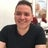#### karimfathy

May. 24, 2015
•Sep. 7, 2015

Total views

3,251

On Slideshare

0

From embeds

0

Number of embeds

217

80

Shares

0